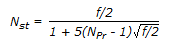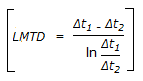# Test: Heat Transfer - 3

## 25 Questions MCQ Test Heat Transfer | Test: Heat Transfer - 3

Description
Attempt Test: Heat Transfer - 3 | 25 questions in 25 minutes | Mock test for Chemical Engineering preparation | Free important questions MCQ to study Heat Transfer for Chemical Engineering Exam | Download free PDF with solutions
QUESTION: 1

Solution:
QUESTION: 2

Solution:
QUESTION: 3

### The dimensionless group in mass transfer that is equivalent to Prandtl number in heat transfer is

Solution:
QUESTION: 4

Finned tube heat exchangers

Solution:
QUESTION: 5

The absorptivity of a grey body at a given temperature __________ with increasing wavelength of radiation.

Solution:
QUESTION: 6

Which one gives the monochromatic emissive power for black body radiation ?

Solution:
QUESTION: 7

For what value of Prandtl number, St = f/2?

Solution:
QUESTION: 8

In a shell and tube heat exchanger,

Solution:
QUESTION: 9

Dietus-Boelter equation used for the determination of heat transfer co-efficient is valid

Solution:
QUESTION: 10

The equation,, corrosponds to __________ analogy.

Solution:
QUESTION: 11

For large heat transfer area requirement, shell and tube heat exchanger is preferred, because it

Solution:
QUESTION: 12

Out of the following four assumptions used in the derivation of the equation for LMTD, which one is subject to the largest deviation in practice ?

Solution:
QUESTION: 13

Heat flux increases with temperature drop beyond the Leiden frost point in the plot of heat flux vs. temperature drop for a boiling liquid, because

Solution:
QUESTION: 14

Resistance to heat flow by conduction is proportional to (where, t & ρ are thickness & density of the material respectively and A = area normal to the direction of heat flow. )

Solution:
QUESTION: 15

Temperature profile in steady state heat transfer is

Solution:
QUESTION: 16

For the same heat load and mass flow rate in the tube side of a shell and tube heat exchanger, one may use multipass on the tube side, because it

Solution:
QUESTION: 17

When vaporisation takes place through a blanketting film of gas, the phenomenon is termed as __________ boiling.

Solution:
QUESTION: 18

Minimum recommended baffle spacing in a shell and tube heat exchanger is about (where, D = shell diameter)

Solution:
QUESTION: 19

Stefan-Boltzman law which describes the radiation heat transfer states that, it is proportional to (where, t = temperature in oC T = absolute temperature in oK )

Solution:
QUESTION: 20

The Nusselt number for fully developed (both thermally and hydrodynamically) laminar flow through a circular pipe, where the wall heat flux is constant, is

Solution:
QUESTION: 21

What is the unit of thermal conductivity ?

Solution:
QUESTION: 22

It is desired to concentrate a 20% salt solution (20 kg of salt in 100 kg of solution) to a 30% salt solution in an evaporator. Consider a feed of 300 kg/min at 30oC. The boiling point of the solution is 110oC, the latent heat of vaporisation is 2100 kJ/kg and the specific heat of the solution is 4 kJ/kg.K. The rate at which the heat has to be supplied in (kJ/min) to the evaporator is

Solution:
QUESTION: 23

It is not preferable to use superheated steam in evaporators, because of its very

Solution:
QUESTION: 24

The thermal radiation emitted by a body is proportional to Tn, where T is its absolute temperature. The value of 'n' is exactly 4 for

Solution:
QUESTION: 25

LMTD for counterflow and prallel flow heat exchanger will be the same, when the

Solution:Use Code STAYHOME200 and get INR 200 additional OFF Use Coupon Code